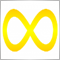# Why do I get OrderSend error on UK100?15980

Why in the following code do I get OrderSend error 131 on UK100 but not on EURUSD?

```// Input variables
input int MagicNumber = 101;
input int Slippage = 10;

input double LotSize = 0.1;
input int StopLoss = 20;
input int TakeProfit = 20;

input int MaPeriod = 200;
input ENUM_MA_METHOD MaMethod = MODE_SMA;
input ENUM_APPLIED_PRICE MaPrice = PRICE_CLOSE;

// Global variables

// OnTick() event handler
void OnTick()   // void doesnt use a return value
{
// Current order counts
int buyCount = 0, sellCount = 0;  // sets object back to 0

for(int order = 0; order <= OrdersTotal() - 1; order++)
{
bool select = OrderSelect(order,SELECT_BY_POS);

if(OrderMagicNumber() == MagicNumber && select == true) // recognises the magicnumber of order recognises magic number of EA realises theres been an order selected by OrderSelect () function
{
else if(OrderType() == OP_SELL) sellCount++;
}
}

// Moving Average and Close price from last bar                Section 3
double close = Close;
double ma = iMA(_Symbol,_Period,MaPeriod,0,MaMethod,MaPrice,1);

// Buy order condition                                     Section 4
{
// Close sell order         OrderTotal() is a function that returns the amount of open orders.
for(int order = 0; order <= OrdersTotal() - 1; order++)    // for loop finds any open orders
{                         // 1      index in order pool
bool select = OrderSelect(order,SELECT_BY_POS);   // selects the open order (Order index or order ticket depending on the second parameter.)(SELECT_BY_POS - index in the order pool)

if(OrderType() == OP_SELL && OrderMagicNumber() == MagicNumber && select == true) // checking its a sell checking magicnumber matches and it has selected an order
{
// Close order
bool closed = OrderClose(OrderTicket(),OrderLots(),Ask,Slippage,clrRed);  // Notice you can input functions written OrderTicket() as parameters
if(closed == true) order--;  // order -- decrements order from 1 to 0 so it will stop iterating
}
}

// Open buy order                                                                                      Section 5
gSellTicket = 0;

if(gBuyTicket > 0 && (StopLoss > 0 || TakeProfit > 0))
{

// Calculate stop loss & take profit
double stopLoss = 0, takeProfit = 0;
if(StopLoss > 0) stopLoss = OrderOpenPrice() - (StopLoss * _Point);
if(TakeProfit > 0) takeProfit = OrderOpenPrice() + (TakeProfit * _Point);

// Verify stop loss & take profit
double stopLevel = MarketInfo(_Symbol,MODE_STOPLEVEL) * _Point;

RefreshRates();
double upperStopLevel = Ask + stopLevel;
double lowerStopLevel = Bid - stopLevel;

if(takeProfit <= upperStopLevel && takeProfit != 0) takeProfit = upperStopLevel + _Point;
if(stopLoss >= lowerStopLevel && stopLoss  != 0) stopLoss = lowerStopLevel - _Point;

// Modify order
}
}

// Sell order condition
if(close < ma && sellCount == 0 && gSellTicket == 0)
{
for(int order = 0; order <= OrdersTotal() - 1; order++)
{
bool select = OrderSelect(order,SELECT_BY_POS);

if(OrderType() == OP_BUY && OrderMagicNumber() == MagicNumber && select == true)
{
// Close order
bool closed = OrderClose(OrderTicket(),OrderLots(),Bid,Slippage,clrRed);

if(closed == true)
{
order--;
}
}
}

// Open sell order
gSellTicket = OrderSend(_Symbol,OP_SELL,LotSize,Bid,Slippage,0,0,"Sell order",MagicNumber,0,clrRed);

// Add stop loss & take profit to Sell order
if(gSellTicket > 0 && (StopLoss > 0 || TakeProfit > 0))
{
bool select = OrderSelect(gSellTicket,SELECT_BY_TICKET);

// Calculate stop loss & take profit
double stopLoss = 0, takeProfit = 0;
if(StopLoss > 0) stopLoss = OrderOpenPrice() + (StopLoss * _Point);
if(TakeProfit > 0) takeProfit = OrderOpenPrice() - (TakeProfit * _Point);

// Verify stop loss & take profit
double stopLevel = MarketInfo(_Symbol,MODE_STOPLEVEL) * _Point;

RefreshRates();
double upperStopLevel = Ask + stopLevel;
double lowerStopLevel = Bid - stopLevel;

if(takeProfit >= lowerStopLevel && takeProfit != 0) takeProfit = lowerStopLevel - _Point;
if(stopLoss <= upperStopLevel && stopLoss != 0) stopLoss = upperStopLevel + _Point;

// Modify order
bool modify = OrderModify(gSellTicket,0,stopLoss,takeProfit,0);
}
}
}```Moderator
20477

Maybe a lot size of 0.1 is not allowed by the broker on UK10015980

GumRai:
Maybe a lot size of 0.1 is not allowed by the broker on UK100
Thanks for letting me realise this GumRai. I see on specifications the minimal lot size is 1.0 for UK100.5

```// Sell order condition
if(close < ma && sellCount == 0 && gSellTicket == 0)
{
for(int order = 0; order <= OrdersTotal() - 1; order++)
{
bool select = OrderSelect(order,SELECT_BY_POS);

if(OrderType() == OP_BUY && OrderMagicNumber() == MagicNumber && select == true)
{
// Close order
bool closed = OrderClose(OrderTicket(),OrderLots(),Bid,Slippage,clrRed);

if(closed == true)
{